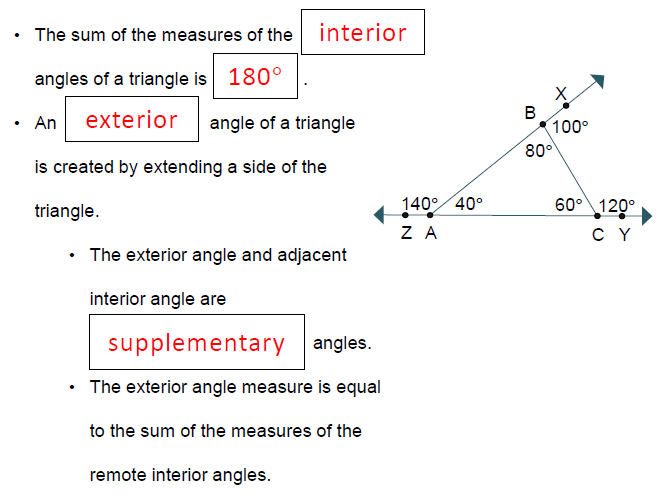# UNIT 4 LESSON 1: TRIANGLE ANGLE THEOREMS

## WORDS TO KNOW:

Here is the link to the flashcards if you need help with terms and definitions.

## ANGLE RELATIONSHIPS REVIEW:Use the diagram of g||h, with transversal f, to answer the questions. A pair of corresponding angles is ______________.

Select all that apply
• A
• B
• C

Angle 2 is _________ angle 8

Select all that apply
• A
• B
• C

A pair of same-side interior angles is _____________.

Select all that apply
• A
• B
• C

## REMEMBER!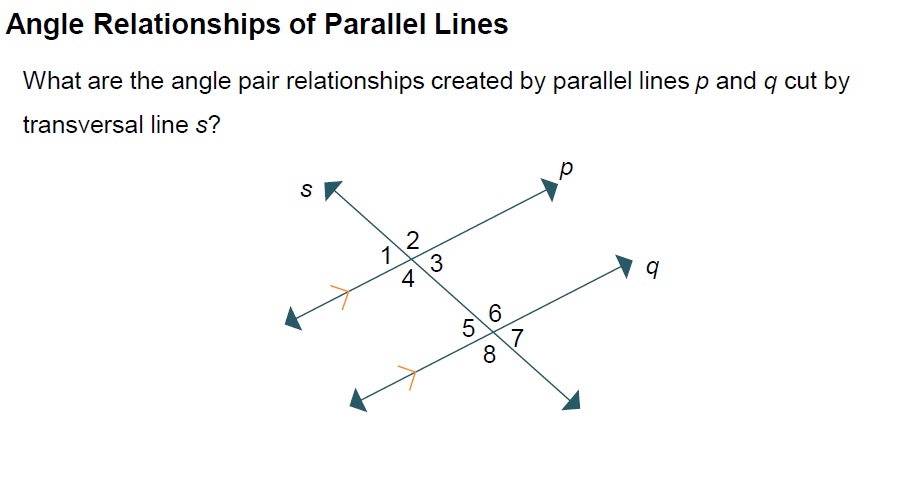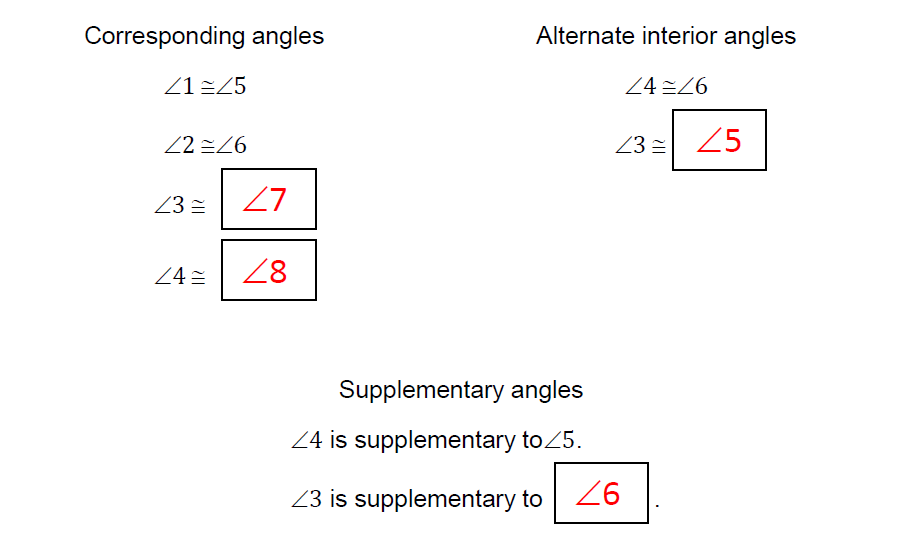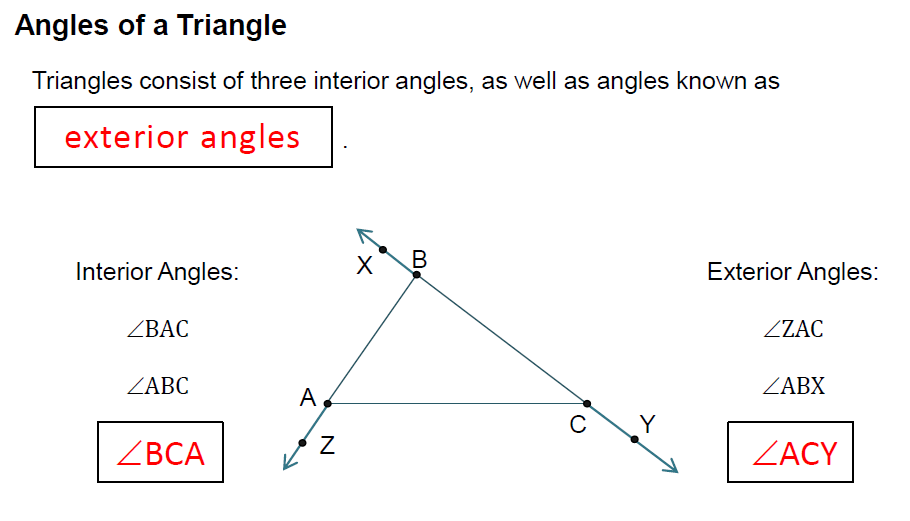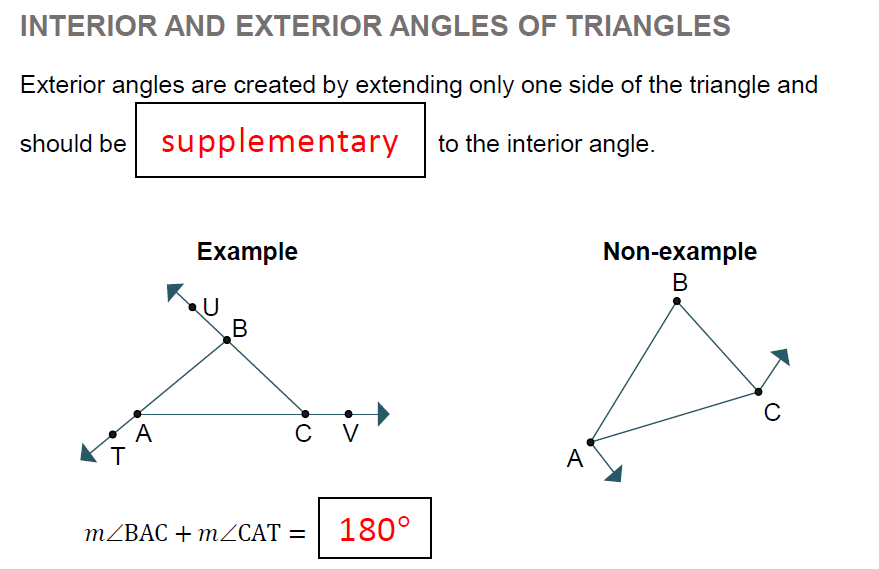Which statements regarding the diagram are true? Check all that apply.

Select all that apply
• A
• B
• C
• D
• E
• F

## INTERIOR AND EXTERIOR ANGLE THEOREMS

Interact with the applet below for a few minutes.   Then, answer the questions that follow.   REMEMBER! An exterior angle is an angle on the outside of a triangle that is a linear pair to the interior angle of the triangle. Be sure to change the locations of the triangle's WHITE VERTICES each time before you drag the slider!!! TYPE YOUR ANSWERS AND CLICK THE CHECK YOUR ANSWER BOX.

## INTERIOR AND EXTERIOR ANGLE THEOREM QUESTIONS:

1. What geometric transformations took place in the applet above for interior angles? CHECK ALL THAT APPLY

Select all that apply
• A
• B
• C
• D
• E

2. When working with the triangle's interior angles, did any of these transformations ﻿change the measures of the blue or green angles?

3. From your observations, what is the sum of the measures of the interior angles of     any triangle?

4. When working with the triangle's exterior angles, did any of these ﻿transformations change the measures of the green or gray angles?

5. From your observations, what is the sum of the measures of the exterior angles of     any triangle?

## ANOTHER WAY TO FIND THE SUMS OF INTERIOR ANGLES OF A TRIANGLE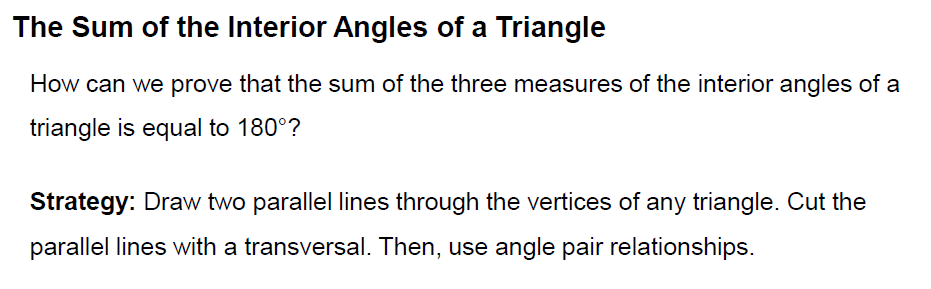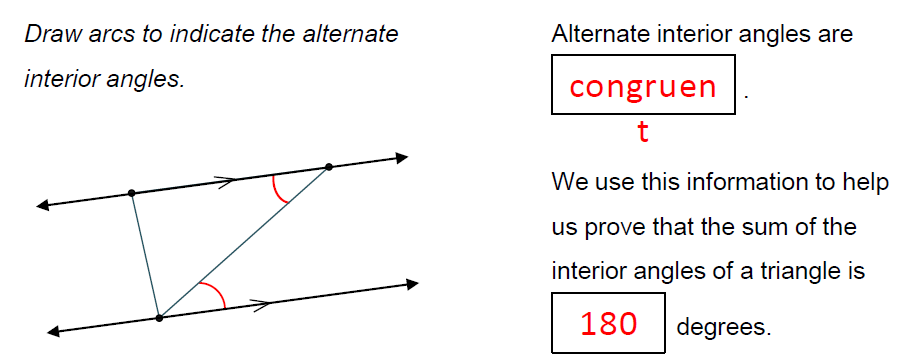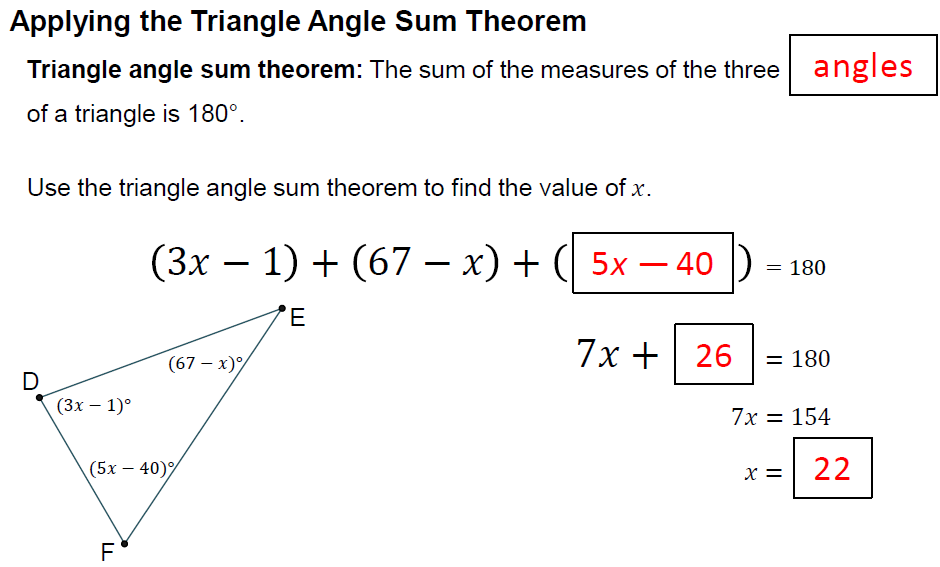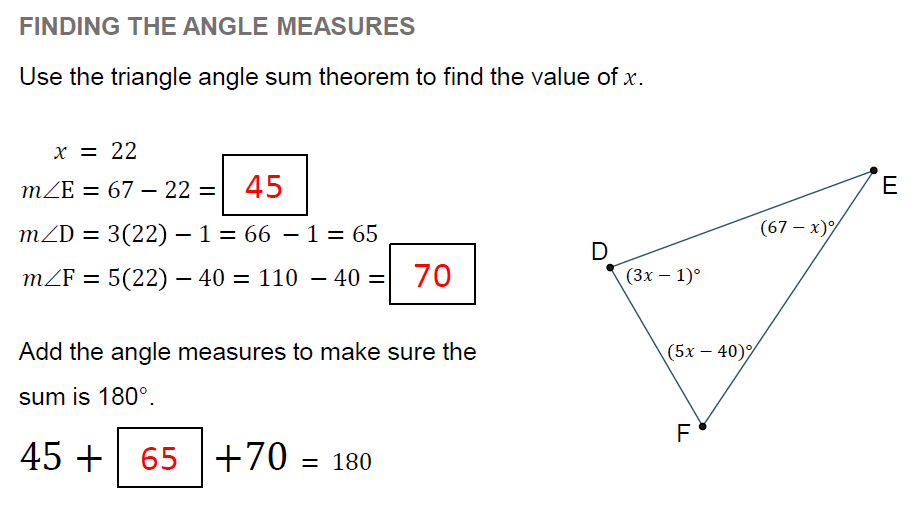Select all that apply
• A
• B
• C
• D

## THIRD ANGLE THEOREM

Move the white vertices to change the shape of the triangle. Decide what the relationship is between the unknown angle and the other two angles.

## THIRD ANGLE THEOREM

6. Describe how can you find the measure of angle C?

We use this information to help us prove that the sum of the interior angles of a triangle is _______ degrees.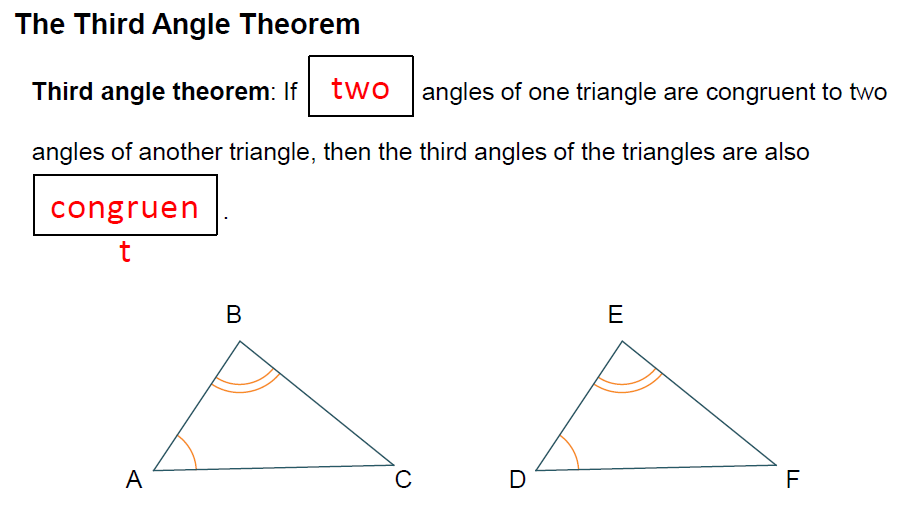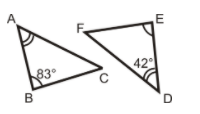Determine the measure of the missing angles.

## EXTERIOR ANGLE THEOREMS

The two interior angles that are not a linear pair with the exterior angle are called REMOTE ANGLES. Move the slider, then move the white vertices to see what happens.

## EXTERIOR ANGLE THEOREM QUESTIONS:

7. What geometric transformations took place in the applet above? CHECK ALL THAT APPLY

Select all that apply
• A
• B
• C
• D
• E

8. As you move the vertices on the applet, what do you notice about the remote ﻿angles and the exterior angle?

9. What is the relationship between the exterior angle and ONE of the remote angles?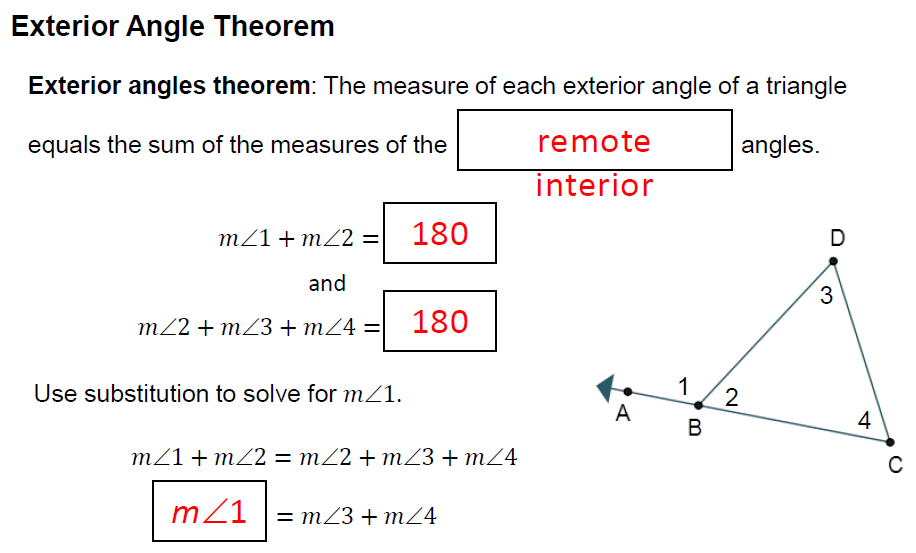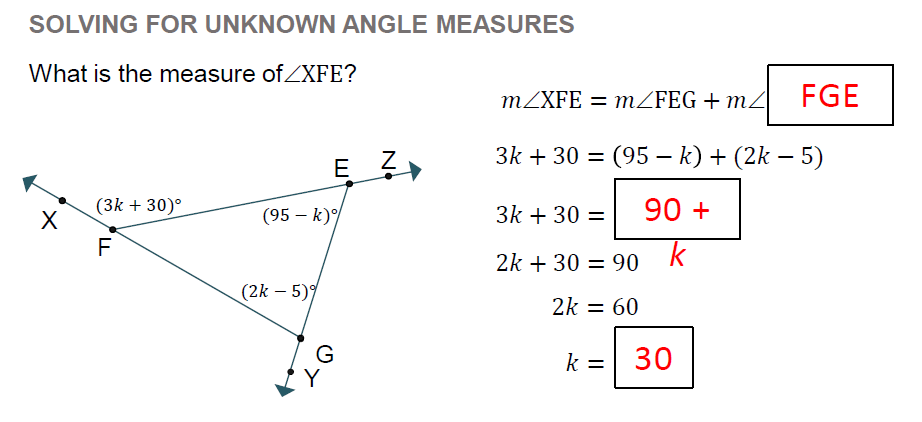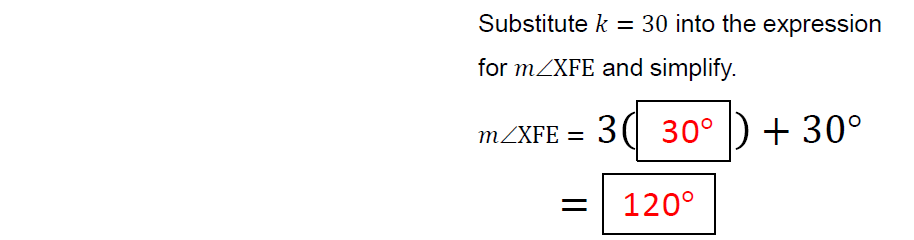What is the measure of ∠XBC?

1. m∠XBC = m∠BAC + m∠BCA
2.  3p – 6 = p + 4 + 84
3.  3p – 6 = p + 88
4.  2p – 6 = 88
5. 2p = 94
m∠XBC = _______ °

## ANALYZING THE RELATIONSHIPS BETWEEN INTERIOR AND EXTERIOR ANGLES OF TRIANGLES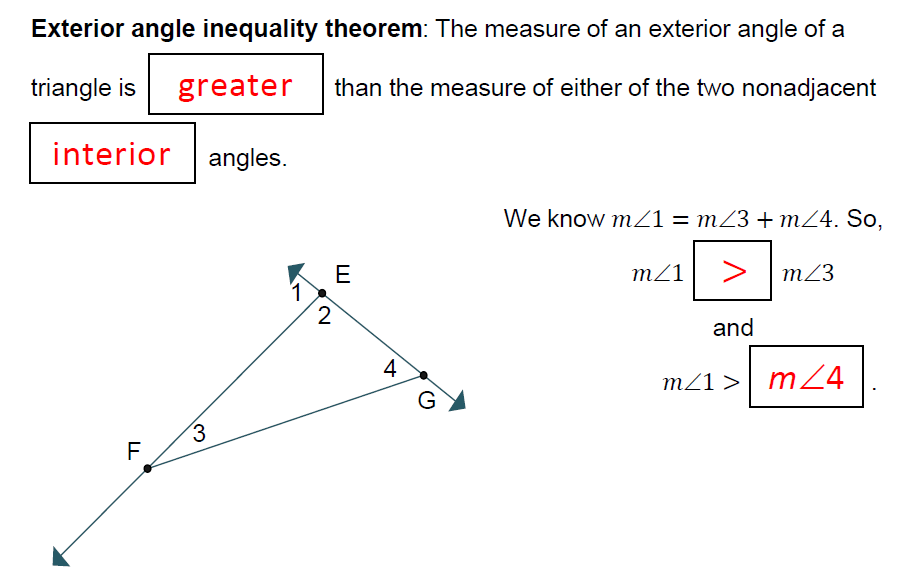Analyze the diagram to complete the statements. The m∠MXN is __________ m∠YZX.

Select all that apply
• A
• B
• C

The m∠LZX is __________ m∠ZYX + m∠YXZ.

Select all that apply
• A
• B
• C

The m∠MYL is ________ 180° − m∠ZYX.

Select all that apply
• A
• B
• C